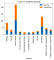# Data exploration made easy — subplots in Matplotlib

Doing more work with less effort is the name of the game in coding. For data scientists, this is a huge advantage to using tools like Python to assist with extracting as much information from data as possible in an efficient way.

Data exploration is one of the early steps of the data science process. During this step, it is important to gain an understanding of the structure and content of the information you are working with. There are functions like .describe() and .info() which make it easy to get cursory summary statistics about numerical data, identify skew, see null values, and identify data types.

Beyond this, it is often helpful to begin to visualize the data. This will also help the analyst to spot outliers and see the shape of the data. A popular Python library for visualization is Matplotlib and pyplot, a collection of functions that make Matplotlib function like MATLAB. Using Matplotlib.pyplot, you can create a number of plots, from box plots to histograms, to begin to paint a picture with the data.

Setting up a basic plot is easy:

`Import matplotlib.pyplot as plt%matplotlib inline`

You write the matplotlib inline to display plots directly in your Jupyter Notebook. For this exercise, we are using data from student test performance at a few high schools.

Here is an example of how you would plot a bar plot to compare the number of students who were at a passing level vs those who were not.

`subjects = list(set(scores['Subject']))passing = []notpassing = []y = {}for i in subjects:    x = scores[scores['Subject'] == i]    passing.append(len(x[x['Passing'] == 'Passing']))    notpassing.append(len(x[x['Passing'] == 'Did not pass']))y['subjects'] = subjectsy['passing'] = passingy['notpassing'] = notpassingdf = pd.DataFrame.from_dict(y)df = df.set_index('subjects')df.plot(kind='bar',stacked=True)plt.title('Count of students passing')plt.show();`

And the output would be:Single bar chart

Now, you could write out a cell of code to make a plot for each of the various comparisons you would like to make. However, that goes against our ideal of doing more for less. This is where the beauty of subplots comes in. With subplots, you are able to generate an array of plots, iterating through data and creating multiple plots at once. This provides insights faster, allowing for deeper insights and more targeted analysis.

To establish subplots, begin by generating the plot figure and axes. You can also specify the number of rows and columns in the array.

`Fig, axs = plt.subplots(n_rows, n_columns, figsize=(14,24))`

You can also specify the figure size when you initialize the plot.

Following the initialization, specify the space you need between plots using the plt.subplots_adjust() function. Wspace and hspace will determine the space between each of the plots on the sides and header.

`plt.subplots_adjust(wspace=0.6, hspace=0.6)`

Next, you would set up your data into manageable lists. I’ve also structured the lists into a dictionary to assist with plot labels.

`schools = list(set(scores['High School']))frpl = list(set(scores['FRPL']))gender = list(set(scores['Gender']))ethnicity = list(set(scores['Ethnicity']))metrics = {'High School': schools, 'FRPL': frpl, 'Gender': gender,            'Ethnicity': ethnicity}`

The way I’ve structured my data in this example, I iterate through my dictionary keys and use them to filter down the primary data set, adding the counts of passing and not passing scores to lists. The lists are then fed into a dictionary, which I then use to construct a dataframe. This is where I begin to construct my subplots.

We can use variables to select the rows and columns of the axis of the subplot. Because I am constructing stacked bar plots, I call two barplots to the same axis position. It is easy enough to then call in the dictionary keys as labels for the plots.

To generate the tick marks, I have generated a set of the values in each of the variables I am using to split the data. I then specify the number of tick marks and the labels by passing the set into the plt.set_xticks and plt.set_xticklabels calls. You can also adjust the font size and rotation for the tick marks.

I have also chosen to plot the percentage of students passing the tests in each of the demographic groups.

`a = 0for i, j in enumerate(metrics.keys()):    passing = []    notpassing = []    y = {}    z = metrics[j]    for k in z:        x = scores[scores[j] == k]        passing.append(len(x[x['Passing'] == 'Passing']))        notpassing.append(len(x[x['Passing'] == 'Did not pass']))            y[j] = z    y['passing'] = passing    y['notpassing'] = notpassing        N = len(passing)    ind = np.arange(N)    ticks = list(range(N))    df = pd.DataFrame.from_dict(y)    df = df.set_index(j)    axs[a,0].bar(ind, df['passing'], width=0.6)    axs[a,0].bar(ind, df['notpassing'], bottom=df.passing,width=0.6)    axs[a,0].set_title('Count of test takers')    axs[a,0].set_xlabel(j)    axs[a,0].set_xticks(ticks)    axs[a,0].set_xticklabels(z, fontdict = {'rotation': 45})        df['passrate'] = (df['passing'] / (df['passing'] + df['notpassing']))*100        axs[a,1].bar(ind, df['passrate'], width=0.6)    axs[a,1].set_title('Percentage of students passing')    axs[a,1].set_xlabel(j)    axs[a,1].set_xticks(ticks)    axs[a,1].set_xticklabels(z, fontdict = {'rotation': 45})    a += 1`

Here is the output:

So with one block of code, I’m able to produce 8 graphs; 4 pairs of informative graphs that tell me quite a bit about the information I’m working with!

Hopefully this provides you with a good introduction to subplots in matplotlib.